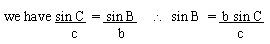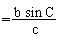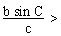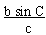Support the Monkey! Tell All your Friends and TeachersHome MonkeyNotes Printable Notes Digital Library Study Guides Study Smart Parents Tips College Planning Test Prep Fun Zone Help / FAQ How to Cite New Title Request

3.4 The Ambiguous Case Of The Law Of Sines

If two sides and one angle opposite to one of them are given, there can be complications in solving triangles. In fact, from the given data, we may determine more than one triangle or perhaps no triangles at all.

Let the angle be C and sides be c and b of triangle ABC given thenHere, R.H.S. is completely known and hence Ð B can be found out.

Also Ð C is given \ ÐA = 1800 - (B + C). Thus triangle ABC is solved.

But when sin Bhas R. H.S. with such values that the triangle can't be com pleted.

Observe,

(I) When Ð C < 900 (acute angle)

(a) If c < b sin C then1 i.e. sin B > 1 which is impossible (as sine c ratio never exceeds 1)

(b) c = b sin C then= 1 i.e. sin B = 1 \ Ð B = 900 i.e triangle ABC is c right triangle.

(c) If c > b sin C then< 1 i.e. sin B < 1. But within the range 00 to 1800 ; there are two values of angles for a sine ratio as sin q = sin (180 - q), of which one is acute and the other is an obtuse. e.g. sin 300 = sin 1500Your browser does not support the IFRAME tag.

But both of these may not be always admissible.

If ÐB < 900 (acute), the triangle ABC is possible as c > b then Ð C > Ð B. But if Ð B > 900 (obtuse) then Ð C will be also obtuse ; this is impossible as there can't be two obtuse angles in a triangle.

If c < b and Ð C is acute then both values of B are admissible . Naturally there will be two values of Ð A and hence two values of a. Hence there are two triangle possible.

(II) When Ð C < 900 (obtuse angle).

Here (1) If c < b then Ð C < Ð B but then B will also become an obtuse angle. It is also impossible.

(2) If c = b then Ð B = Ð C. Hence Ð C is obtuse makes Ð B obtuse too which again impossible.

(3) If c > b then Ð C > Ð B. But again there are two possibilities (i) Ð B is acute (ii) and Ð B is obtuse.

If Ð B is acute the triangle is possible and when Ð B is obtuse the triangle is impossible. For the obtained values of the elements when there is ambiguity (i.e. we are unable to draw such a triangle) to determine the triangle ; it is called an 'ambiguous case'.

Index

3. 1 Solving Right Triangles
3. 2 Law of Cosines
3. 3 Law of Sines
3. 4 The Ambiguous Case of Law of Sines
3. 5 Areas of Triangles
Supplementary Problems

Chapter 4Search: All Products Books Popular Music Classical Music Video DVD Toys & Games Electronics Software Tools & Hardware Outdoor Living Kitchen & Housewares Camera & Photo Cell Phones Keywords: